# A New Framework Containing Convolution and Pooling Circuits for Image Processing and Deep Learning Applications with Quantum Computing Implementation

A New Framework Containing Convolution and Pooling Circuits for Image Processing and Deep Learning Applications with Quantum Computing Implementation
Department of Computer Engineering, Fırat University, Elazig 23119, Turkey

Corresponding Author Email:
h.yetis@firat.edu.tr
Page:
501-512
|
DOI:
https://doi.org/10.18280/ts.390212
24 February 2022
|
Accepted:
10 April 2022
|
Published:
30 April 2022
| Citation

OPEN ACCESS

Abstract:

The resource need for deep learning and quantum computers' high computing power potential encourage collaboration between the two fields. Today, variational quantum circuits are used to perform the convolution operation with quantum computing. However, the results produced by variational circuits do not show a direct resemblance to the classical convolution operation. Because classical data is encoded into quantum data with their exact values in value-encoded methods, in contrast to variational quantum circuits, arithmetical operations can be applied with high accuracy. In this study, value-encoded quantum circuits for convolution and pooling operations are proposed to apply deep learning in quantum computers in a traditional and proven way. To construct the convolution and pooling operations, some modules such as addition, multiplication, division, and comparison are created. In addition, a window-based framework for quantum image processing applications is proposed. The generated convolution and pooling circuits are simulated on the IBM QISKIT simulator in parallel thanks to the proposed framework. The obtained results are verified by the expected results. Due to the limitations of quantum simulators and computers in the NISQ era, the used grayscale images are resized to 8x8 and the resolution of the images is reduced to 3 qubits. With developing the quantum technologies, the proposed approach can be applied for bigger and higher resolution images. Although the proposed method causes more qubit usage and circuit depth compared to variational convolutional circuits, the results they produce are exactly the same as the classical convolution process.

Keywords:

quantum computing, quantum deep learning, quantum information processing

1. Introduction

Today, deep learning (DL) is used in every field from self-driving cars to artificial intelligence (AI) that can write poems and draw pictures [1-3]. Besides its success, the high computatiınal power they need is one of the biggest disadvantages of DL. A DL network can run over and over for the same input data, and it needs a lot of different data . Since training a DL network from scratch is very difficult and requires very powerful computers, today's DL-related works are usually carried out through pre-trained networks .

DL consists of steps such as convolution, pooling, and a fully-connected layer. The steps of DL are given in Figure 1 . Today, the most commonly used DL networks in the literature are CNN (Convolutional Neural Network), RNN (Recurrent Neural Network), and LSTM (Long Short-Term Memory) . However, convolution and pooling operators used in the feature extraction phase are common to each .

## 1.png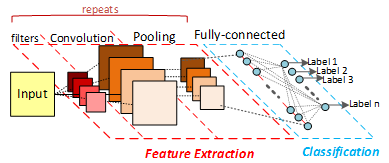Figure 1. General DL steps

Supercomputers are known as the most powerful computers today. For example, the Fugako supercomputer, which is on the list of the best 500 supercomputers in 2020, consumes up to 30-40 MWh of power [9, 10]. In contrast, quantum computers that are reported to be exponentially faster than a supercomputer, have a power consumption of only 25 kWh [11, 12]. Quantum computers offer significant potential for certain problems in terms of processing power. DL and Quantum Computing (QC) are closely related in terms of complementing each other .

1.1 Motivation

In this study, the quantum computation models of convolution, and pooling which are used in DL networks, have been carried out in a classical way that differs from the literature. In literature, the studies about quantum machine learning are focused on variational quantum circuits which aim to emulate the target function within an arbitrarily small error with fewer circuit elements . The main motivation of the study is to model DL steps exactly in their original form in a quantum computing environment. Our supportive motivations are listed below:

• Training of DL networks is a time-consuming process that requires high-performance computing (HPC).
• Existing DL networks are usually pre-trained networks. It is predicted that the success of a DL network trained from scratch will be more than that of a pre-trained one .
• Lack of computational power is one of the biggest obstacles to the training of DL networks.
• QC has high computing power with low power consumption [9, 10].
• With the use of QC in DL, the training of DL networks from scratch will be easier and better solutions can be achieved by trial and error experiments.
• Implementation of DL networks in a proven classical way ensures the results with exact high accuracy, as opposed to the possible results of variational circuits.
• With the practical use of QC in AI, a new era for AI will open its doors [15, 16].

1.2 Background

Since QC is based on quantum physics, it differs fundamentally from binary computing. This difference is also seen in the basic gates used in circuit creation. The basic quantum gates are Hadamard, Pauli-X (NOT), Pauli-Y, Pauli-Z, Phase, pi/8, CNOT, Swap, and Toffoli gates . In QC, the CNOT, and Toffoli gates are used to make the qubits entangled. The Hadamard gate is a frequently used gate to puts the inputs into superposition. Thanks to the superposition principle, quantum computers are capable of parallel processing . The basic quantum gates and notations used in the study are given in Table 1. For detailed information about quantum gates, readers are encouraged to read [17, 19].

Table 1. Essential quantum gates used in this study

Gate Name

Symbol

Mission

Pauli-X (NOT)

## a.png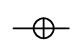Take the inverse of the qubit

## b.png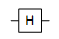Puts the inputs in superposition

Controlled Not (CNOT)

## c.png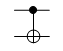Take the inverse of the target qubit only if the control qubit is 1

Multi Controlled Toffoli

(MCT)

## d.png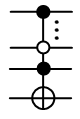Take the inverse of the target qubit only if the control qubits are satisfied the condition (filled circle means 1, hollow circle means 0). If it has only 2 filled control qubits, the gate is called Toffoli.

Controlled Swap

## e.png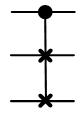Swap the target qubits only if the control qubit is 1

Quantum algorithms, or circuits, can be developed by using basic quantum gates . Early examples of these algorithms are the Deutch, Shor, and Grover algorithms, which are developed in the 1990s [21-23]. It has been shown that with these basic algorithms, quantum computing can be performed faster than binary computing. However, it is not easy to run the developed algorithms on hardware. Although Shor's algorithm was developed in 1994, it is implemented for the first time on a real quantum computer in 2001 for factoring the number 15 . It is seen that quantum algorithms were developed before suitable quantum computers and suitable quantum computers become available much later.

1.3 Literature

Many studies aim to use the advantage of QC in different machine learning methods [25-28]. DL is a machine learning method, based on Artificial Neural Network (ANN), and even more on perceptrons. There are also many studies in the literature on the realization of perceptrons with quantum circuits [29-32]. Apart from these, a pure literature summary of recent studies on quantum DL is given in Table 2 with their strengths and weaknesses. As seen in the table some studies aim to realize convolution and pooling steps with variational quantum circuits. The main contribution of the paper is implementing the DL steps classically. It has not been proven how the performances obtained as a result of small size and limited trials of variational circuits will work in big data. The study guarantees that the results obtained with the study will be the same as the results obtained with the proven traditional DL network.

Table 2. Recent studies in the literature

 Ref. Year Main Contribution Difference from our study  2021 A novel image recognition framework is proposed for optical quantum computers. The proposed framework is for optical quantum computers.  2021 It has been suggested to perform the convolution process more effectively using the QRAM model. It has been suggested that the QRAM model be used in the variational convolution process.  2020 Hybrid machine learning tools have been created and made available for the Tensorflow library. It allows modeling of variational quantum circuits for convolution and pooling.  2020 Variational circuits have been proposed for quantum DL. Variational circuits, which have not been proven for big data, are used.  2020 Algorithms using the QRAM model have been proposed for the convolution process The proposed algorithms are realized by serialization instead of iterative convolution. This requires a higher number of qubits.  2020 It has been suggested Quantum CNN algorithm be used in breast cancer diagnosis through images. Variational circuits, which have not been proven for big data, are used.  2020 Classification has been made on the MNIST data set using the Tensorflow quantum platform. Variational circuits, which have not been proven for big data, are used.  2020 Quantum circuits with 2, 3, and 4 window sizes have been proposed for the binary convolution process. The proposed quantum circuit is for binary inputs. Only 2,3,4 dimensional circuits are handled.  2019 Variational circuits for quantum convolution and pooling have been proposed. Variational circuits, which have not been proven for big data, are used.
2. Materials and Methods

In this section, used quantum image representation model and quantum modules such as addition, multiplication, comparison, and sorting are explained.

2.1 Quantum image representation

Images are frequently used as input data in DL methods. The storage of an image in conventional computers is carried out in matrix form. There are different color representation methods for storing images such as grayscale images. Grayscale images are capable of expressing each pixel in a single color without disturbing the contours of the picture. To set the DL steps to work on quantum computers, the input data must first be transformed into a quantum representation model. Some approaches such as qubit encoding, amplitude encoding, and feature encoding are used for encoding the classical data to quantum data. Different models such as NEQR (Novel Enhanced Quantum Representation), FRQI (Flexible Representation of Quantum Images), SQR (Simple Quantum Representation), etc. are proposed for quantum image representation . In the study, the NEQR model, which is a qubit encoding approach, is preferred to apply arithmetic operators easily and work with gray-level images . According to NEQR, a pixel is stored as shown in (1), where $\left|C_{Y X}\right\rangle$ is color, $|Y\rangle$, and $|X\rangle$  are coordinates. Considering the color depth of $\left|C_{Y X}\right\rangle$ is q qubits, the colors in the range of [0- $2^{q-1}$] can be coded as in classical computers. The NEQR representation for the $2^{n} x 2^{n}$ image is given in (1). A sample image and its representation for n=1, are given in Table 3.

$|I\rangle=\frac{1}{2^{n}} \sum_{Y=0}^{2^{n}-1} \sum_{X=0}^{2^{n}-1}\left|C_{Y X}\right\rangle|Y\rangle|X\rangle$     (1)

Table 3. Sample NEQR model for n=1

Sample Image

Representation

## s.png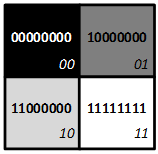$I=\left[\begin{array}{cc}0 & 128 \\ 192 & 255\end{array}\right]$

## ss.png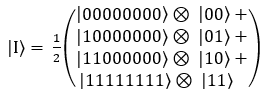2.2 Basic modules for quantum circuits

This section introduces the basic modules required for DL convolution and pooling.

To create the adder module, half and full adders circuits are used. While the half adder takes two inputs, the full adder considers the previous carry bit as well as the input values. The sum of qubits is actualized by taking the inverse of the ancilla qubit, which is 0 at the beginning if one of the inputs is 1. When both 2 inputs are 1, then the sum qubit is 0 and the carry qubit is 1. In this way, the truth table of the adder is ensured. Half and full adder quantum circuits are given in Figure 2. While the whole image belongs to the full adder, the dotted rectangle represents the half adder circuit.

## 2.png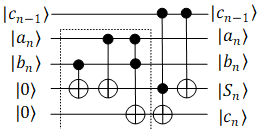Figure 2. Quantum full adder circuit where the dotted line is for a half adder, $a_{n}$ and $b_{n}$ are $\mathrm{n}^{\text {th }}$ bits of inputs, $c_{n-1}$ is input carry bit, $c_{n}$ is output carry bit, and $S_{n}$ is the result bit

Using these adders sequentially, an adder circuit with n qubit is created . In the adder circuit, the output must be one qubit more than the inputs. The temporary carry bits are garbage qubits, which are not necessary for output. There are quantum adder circuit designs with extra garbage and carry bits . But for the operations such as convolution and pooling, the extra qubits make it harder to build the circuit. The suggested module to perform quantum addition without using extra qubits can be seen in Figure 3.a, where $|a\rangle$ and $|b\rangle$ are the input states. The output $|\mathrm{a}+b\rangle$ is overwritten on input $|\mathrm{b}\rangle$. For large sums that cannot be expressed in n qubits, one more qubit must be added to the output. If the sum can be expressed with n qubit, there is no need extra (carry) qubit. The quantum adder circuit with one carry qubit is given in Figure 3.b for n=3 .

## 3.png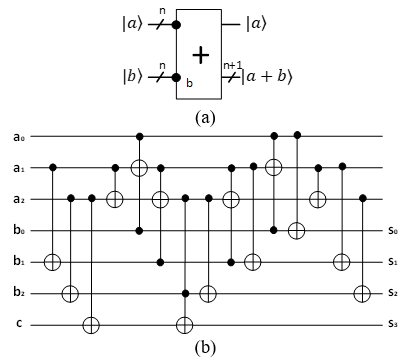Figure 3. Quantum self-adder module and circuit a) Adder quantum circuit module where $|a\rangle$ and $|b\rangle$ are the inputs with n qubits, $|\mathrm{a}+b\rangle$ is the output with $\mathrm{n}+1$ qubits b) 3 -qubit selfadder circuit, the output is stored in $\mathrm{S}_{3} \mathrm{~S}_{2} \mathrm{~S}_{1} \mathrm{~S}_{0}$

2.2.2 Plus 1 and minus 1 modules

## 4.png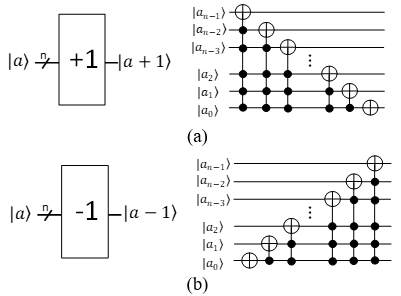Figure 4. Plus 1 and Minus 1 quantum circuits. a) Plus 1 circuit, b) Minus 1 circuit

Quantum plus 1 and minus 1 operators are important for fetching a window from the encoded image. For the plus 1 module, all qubits are checked, starting with the most weighted qubit first. If all subsequent qubits are 1, the inverse of the current qubit is taken. At the end of the process, the last significant qubit a0 is inverted. The plus 1 quantum circuit is given in Figure 4.a . In reversible circuits, a state can be reverted by applying the operators in reverse order. So minus 1 module can be written as reverse order plus 1 module. The minus 1 quantum circuit is given in Figure 4.b.

2.2.3 Multiplication module

Multiplying binary numbers can be calculated by the sum of shifted numbers. Since modeling the multiplication process requires parameterized circuits and loops, 2, and 4 multiplication modules are proposed . Multiplying a number with the power of 2 can be practically provided by shifting the qubits and giving the last qubit as 0 . By considering the possibility of the original inputs being used later by other circuits, extra qubits should be used. The modulus of multiplication by 2 and 4 are given in Figure 5 . The dotted lines represent the circuits with no carry (ancilla) qubits.

## 5.pngFigure 5. Quantum multiplication modules for 2 and 4 . a) The quantum x2 module and its circuit, where $|a\rangle$ is input, $|a x 2\rangle$ is output. b) The quantum x4 module and its circuit, where $|a\rangle$ is input, $|\mathrm{a} x 4\rangle$ is output

2.2.4 Division module

In binary numbers, dividing by the power of 2 can be accomplished by shifting the bit right. Assume that the number will be divided by 2m. Then, the last m qubit gives the remaining, and the number obtained by shifting the input m times right gives the output. Unlike the multiplication module, the direction of the shifting changes. Moreover, if n qubits are used at the input, (n-m) qubit is obtained as the output. The quantum division modules are given in Figure 6 .

## 6.png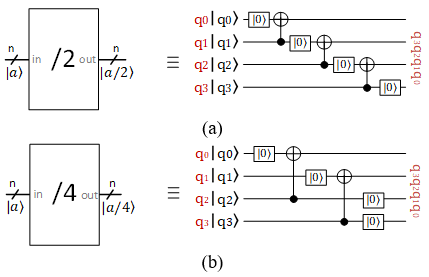Figure 6. Quantum division modules for 2 and 4. a) The quantum /2 module and its circuit, where $|a\rangle$ is input, $|\mathrm{a} / 2\rangle$ is output. b) The quantum /4 module and its circuit, where $|a\rangle$ is input, $|\mathrm{a} / 4\rangle$ is output

2.2.5 Comparison module

A circuit for quantum comparison is proposed by Li in the literature . In this study, a new quantum comparison circuit is proposed by optimizing . With the proposed circuit, it is aimed to increase the applicability of the comparison circuit by using fewer qubits and gates. The proposed comparison circuit takes 2 n qubit inputs and compares them. The proposed circuit and modular presentation are given in Figure 7. The output of the proposed comparison circuit is as given in (2).

$e_{1} e_{0}= \begin{cases}00, & \text { if } x=y \\ 10, & \text { if } x>y \\ 01, & \text { if } x<y \\ 11, & \text { never }\end{cases}$    (2)

## 7.png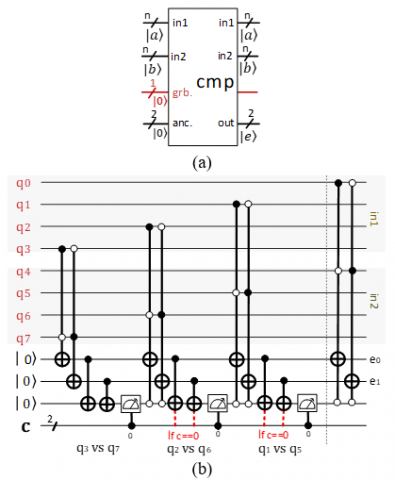Figure 7. The proposed quantum compare module. a) compare module, b) open form of the module for 4 qubits, where $q_{0}-q_{3}$ refers to input a, $q_{4}-q_{7}$ refers to input $b, a_{8}, q_{9}$ refers to ancilla or output, $q_{10}$ refers to garbage qubits

2.2.6 Controlled swap module

In the controlled swap circuit, if the control bit is 1, the inputs switch places, if it is 0, the inputs are output in the same order. This circuit requires an extra control qubit. The controlled swap module is given in Figure 8 . If the first output is a, the second output must be b; if the first output is b, the second output must be a.

## 8.png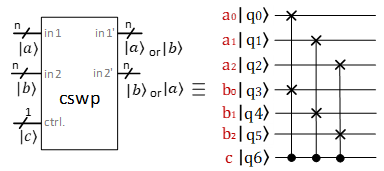Figure 8. Controlled swap module, where c is the control bit, a and b are the n qubit inputs to be swapped. If the control bit is 1, then in1'=b, and in2'=a; otherwise: in1'=a, and in2'=b

2.2.7 Sorting module

Comparison and controlled swapping circuits are required for sorting. The sorting module is given in Figure 9 . If the small value is desired to stay on top, the control qubit must be connected to $e_{0}$. If the large value is desired to be at the top, the control qubit must be connected to $e_{1}$.

## 10.png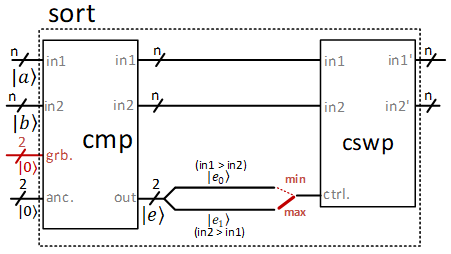Figure 9. Sort module which uses compare and controlled swap sub-modules, where a and b are the n qubit inputs to be sorted. If output $e_{0}$  of compare module is connected to the controlled swap control bit, then in1'  is the minimum in2'  is the maximum; otherwise in1'  is the maximum, in2'  is the minimum

2.2.8 Limit module

The limit circuit ensures that the output takes the max value when it exceeds the max. The limit circuit with max value 7 (lim8) is given in Figure 10, and the output is given in (3). If the original output is not wanted to be collapsed, the corresponding qubit is copied to another qubit by CNOT, and the qubit is collapsed.

$f(x)=\left\{\begin{array}{cl}a_{2} a_{1} a_{0}, & x<8 \\ 111, & \text { else }\end{array}\right.$     (3)

## 1010.png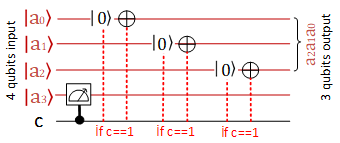Figure 10. Limit circuit for 3 qubits. If $a_{3}$ qubit is 1 (in other words if the number exceeds binary 111), then the other qubits are set to 1 (in other words the number set to binary 111)

3. The Proposed Method

This section shows how to perform operations such as convolution, and pooling quantum operations with the proposed framework.

3.1 Convolution

The convolution process is one of the main operations that makes DL different from other machine learning methods. The convolution process is carried out as given in Figure 11 and equation (4). As can be seen from the equation, the convolution process can be defined as the sum of dot products. So, it is possible to model a quantum convolution circuit with multiplication and addition modules.

## 11.png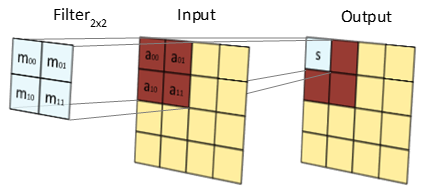Figure 11. Example classical convolution process

$s=\sum_{i=0}^{n} \sum_{j=0}^{n} m_{i j} \cdot a_{i j}$      (4)

Designing a quantum multiplier circuit is very difficult at this stage of quantum computers. For quantum multiplication, it is necessary to develop a cyclic working structure with quantum circuit models that can take parameters or have a memory unit. For the same reason, it is hard to train DL networks. But multiplication or division with the power of 2 is easy to implement by shifting qubits. By using these modules, the convolution operation is applied. Figure 12 shows a 2x2 window and sample masks to be applied to this window. The quantum model circuit required for the application of the masks in between Figure 12.b and Figure 12.e are given in between Figure 13.a and Figure 13.d. The extended version of Figure 13.d is given in Figure 14.

## 12.png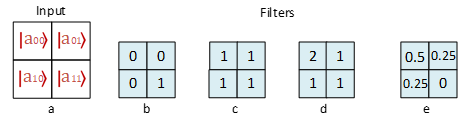Figure 12. Example input and filters for convolution

Figure 14 is obtained by changing the modules in Figure 13.d with their quantum circuit equivalent. Each module is separated by dashed lines. Since the weight corresponding to $\left|a_{11}\right\rangle$ in the original filter is 0, this value is not used in creating the convolution circuit. In the example convolution operation in Figure 14, it is assumed that each of the inputs has 3 qubits (n=3). It can be obtained the circuits with a larger number of inputs and qubits by properly adapting the modules. In the circuit, firstly, input $\left|a_{00}\right\rangle$ is divided by 2, and inputs $\left|a_{01}\right\rangle$ and $\left|a_{10}\right\rangle$ are divided by 4. The division is actualized by bit shifting. It should be noted that the division operation is performed by rounding to lower digits. After the division, the basic quantum circuit equivalents of the addition modules are written. First, $\left|a_{00}\right\rangle$ and $\left|a_{01}\right\rangle$ are added together and written to $\left|a_{01}\right\rangle$. Then $\left|a_{01}\right\rangle$ and $\left|a_{10}\right\rangle$ are added together and written on $\left|a_{10}\right\rangle$. Here in the example, since the sum of the weights is 1, the carry qubit is not required. At the end of the process, the result in $\left|a_{10}\right\rangle$ is copied to the output qubits S.

3.2 Pooling

Pooling is the process of eliminating other pixels by making a selection in a window. Common pooling methods in DL are min pooling, max pooling, mean pooling, and average pooling. According to min pooling, by choosing the smallest value in a window, other values are ignored. According to max pooling, the highest value is chosen. Mean pooling is performed by sorting the data in the window and selecting the value in the middle. Average pooling is done by averaging the values.

3.2.1 Min / Max / Mean Pooling

Min / Max / Mean pooling is performed using sorting circuits. The quantum circuit required for full sorting operation is given in Figure 15 [46, 49]. But for min pooling, it will be sufficient to apply the sequential sorting modules once. That is, only the first part, which calculates in1’, is satisfying. With the change of the control bit given to the sorting circuits, the circuit will work reversely and bring the max element to in1’. If we want to apply mean pooling, we look for in(k/2)’ output. Once the in(k/2)’ output is determined, there is no need to continue sorting. Therefore, (k-1) sorting modules are required for min and max-pooling. The number of sorting modules that should be used for mean pooling is calculated as $\sum_{i=\left|\frac{k}{2}\right|}^{k-1}(i)$, where k is the number of inputs. The quantum circuit for max pooling is given in Figure 16.

## 13.png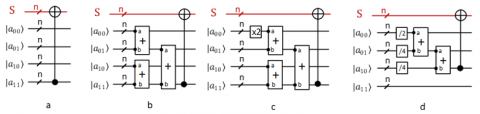Figure 13. Convolution model circuits for filters in Figure 12. a) the circuit for filter Figure 12.b, b) the circuit for filter Figure 12.c, c) the circuit for filter Figure 12.d, d) the circuit for filter Figure 12.e

## 14.png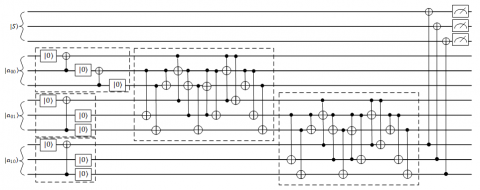Figure 14. Extended version of the model circuit given in Figure 13.d for 3-qubit inputs

## 15.pngFigure 15. Model circuit for min/max/mean pooling

The circuit given in Figure 16 is generated for 4 inputs with 3 qubits. Sorting modules are obtained by sequential use of comparison and controlled swap modules. By using sorting modules in the circuit, it is ensured that the maximum value is moved to the top. In Figure 7, $e_{0}$ and $e_{1}$ qubits are shown for the comparison module. As stated in (2), when the second input is bigger, then $e_{1}=1$. So, only $e_{1}$ qubit connections are done. As a result, when the second input is bigger, controlled swap is actualized. For the quantum min pooling circuit, $e_{0}$ is used instead of $e_{1}$. Each sorting module is shown with dashed lines. The ancilla ($e_{1}$) and garbage qubits are reset after each sorting operation and can be used in the next sorting operation. The garbage qubit is an intermediate qubit whose result is negligible and used to perform calculations. The c in the figure is the classical bit and is used for the measurement result. By using common these extra qubits, the max pooling circuit needs only 2 qubits except for the inputs. In other words, for min/max/mean pooling, $m * n+2$ qubits are required, where m is the number of inputs, and n is the qubit length of inputs.

## 16.pngFigure 16. Max pooling quantum circuit for 4 inputs, where each input is 3-qubit

3.2.2 Average pooling

For practical application of the proposed quantum average pooling circuit, the window size should be the power of 2. For average pooling, the sum of the inputs is calculated first. Then, the numbers are averaged with the help of the division module. An example average pooling circuit for a scenario with 4 inputs is given in Figure 17. The output of the circuit gives the new pixel value.

## 17.png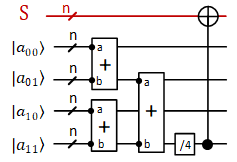Figure 17. Quantum circuit for average pooling

3.3 The proposed framework for image processing

In the previous sections, convolution and pooling circuits have been proposed that take the elements in a window as input. However, for operations such as convolution and pooling to take place in a completely quantum environment, the elements corresponding to the window must first be fetched from the encoded input. In this way, it is possible to perform operations on the entire input image in parallel. In this section, a framework is proposed to fetch neighboring pixels of the relevant pixel according to the window size. In the proposed framework, it requires the input image to repeat as many as the number of non-0 elements in the mask. To fetch the elements, the increment/decrement operations of the relevant coordinates are performed before each repeated image. The encoded image is transferred to qubits holding the window's element at the relevant index each time. The suggested framework for a case with 4 window sizes is given in Figure 18, where I is the encoded image, S is the output, and a00, a01, a10, and a11 are the elements in the window.

## 18.png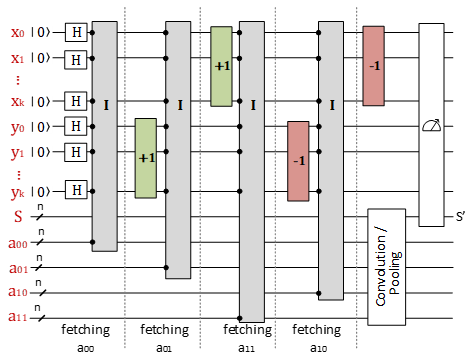Figure 18. The proposed framework for fetching windows, for a case with 4 window sizes, where S' is the result

4. Simulation Results

The proposed methods are tested on a Windows computer with i7 6220M processor, 12 GB RAM, and SSD. The method has been applied in the simulation environment because the proposed methods require lots of qubits, and quantum computers in the NISQ era are not robust for such big circuits [50, 51]. In the study, firstly, quantum encoding is performed to convert classical data to quantum data. NEQR model, which is developed for representing grayscale images, is used for quantum image encoding. According to this model, images are encoded with x, and y coordinates and their real values. In this way, the necessary arithmetic operations can be applied to inputs easily. Then, by using the proposed framework structure, a 2x2 window is fetched from the encoded image. As shown in the proposed framework, parallelization is performed by applying the Hadamard gate to the coordinate inputs. In this way, it is ensured that all windows are fetched at the same time. Finally, sample convolution circuits were created with the help of the given modules and added to the circuit. The qubits from which the results were collected were copied to the output qubit, and the coordinate and output qubits were observed together.

Because of the limitations and performance of the Qisqit simulators, the input images were reduced to 8x8 size with 3 qubit resolution to test the proposed approach. A Matlab code is written which encodes the input image into quantum data. MCT gates are utilized for encoding images, and no optimization method is used. The proposed modules are adopted for 3 qubit color depth, and the circuits are obtained. Because the used images are resized 8x8 (23x23), the pixel coordinates are represented by 6 qubits (3 for x, and 3 for y). The created modules are coded in the IBM QISKIT Quantum Lab environment and tested for all input pairs. The accuracies of modules are checked. QISKIT aer_simulator backend is used for all simulations. To demonstrate the steps of value-encoded quantum convolution, “cameraman.tif” is used. The reduced image is given in Figure 19.a. The matrix form of the image is given in Figure 19.b. To encode this image, the image should be represented by binary numbers. The binary representation of the matrix is given in Figure 20.a. The binary indices in Figure 20.a should give the output in the relevant cell. For example, if x is 000 and y is 000, then the output should be 101. The quantum circuit for encoding the image is given in Figure 20.b. First dotted rectangle is used for encoding (x, y) = (000,000) indices. So, all the controls are negative controls, which are active when the control qubit is 0. Because the data should be 101, the first and last qubits of a00 are reversed, in another word they are set to 1. The process is done for all the cells.

## 19.png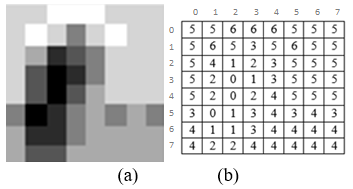Figure 19. The example input image a) cameraman.tif with the size of 8x8 and resolution of 3 qubits, b) matrix form of the image

To calculate the histogram such encoded image, Hadamard gate should be applied on all x and y qubits. And all qubits should be measured. In our example, we measure the qubits in reverse order. So the measurements give the outputs formed in x2x1x0y2y1y0a2a1a0. The histogram of the encoded image is given in Figure 21 for 512 shots. For example, the output 000000101 means there is 101 (last 3 qubits) in the cell indexed by 000 (first 3 qubits), and 000 (second 3 qubits). The measurements done in this way, give us the input indices and output value independently from probabilities. The higher the shots, the more the probabilities tend to be the same. So we don’t care about the probability values.

After the input image is encoded, the circuit must be created with the help of the proposed framework. The generated quantum circuit is given in Figure 22. In the figure, the last dotted rectangle represents the operations formed according to the mask. Here, we design the circuit for the mask in Figure 12.e. The other dotted rectangle represents the encoded images. Gray one is not used and necessary for this mask because the value of a11 is zero in this case. When the image is encoded for the first time, the current index value is reflected in the output. When we increase the X value by one and project the image to other outputs, we get the value to the right of the pixel (a01). When we increase it one more time, we get the element in the lower right corner (a11). When we subtract one from X, the element below (a10) is obtained. In the end, 1 must be subtracted from y to return to the index (a00) again. This is important for proper measurement. In this way, the elements inside the mask window are fetched. Then, using the circuits suggested in the above sections, the relevant convolution or pooling circuits are generated and implemented. Measurement is done after copying the final output to the S qubit. By applying the Hadamard gate to the inputs, a parallel calculation is performed for all the x and y indices at the same time.

The result obtained by running 512 shots of the circuit in Figure 22, is given in Figure 23. Similar to the graph given in Figure 20, the first 6 qubits give the x and y indices, and the last three qubits give the convolution result. Modules such as /2 and /4 use "floor" for rounding. For example, the result of 7/4 is equal to 1. Therefore, it should be taken into account that the calculations are made in this way. By interpreting the obtained results and transforming them into images, Figure 24 appears.

The same procedure is applied for other Matlab built-in demo images for different masks with window sizes 2x2 as shown in Table 4. The results produced by quantum circuits are compared with the expected results. All of the images are as expected. Because the sum of the weights in the used masks is 1, no extra qubit is needed for operations such as sum. With the development of quantum technologies, the proposed method can be applied to images with higher size and color resolutions.

## 20.png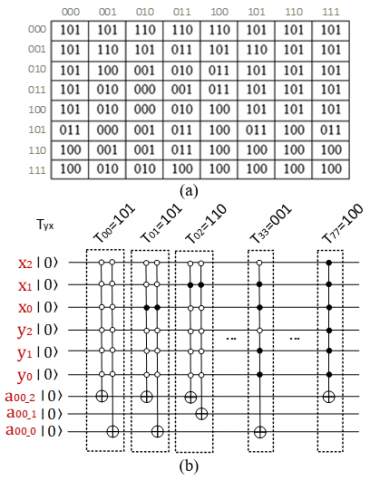Figure 20. Encoding of input a) Binary matrix form of input, b) Quantum circuit for encoding the image

## 21.png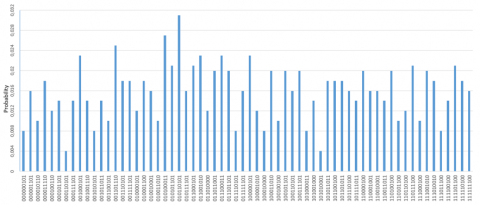Figure 21. Histogram of 3-qubit 8x8 cameraman.tif for 512 shots. The first 3 qubits represent the x coordinate, the second 3 qubits represent the y coordinate, and the last 3 qubits represent the color value

## 22.png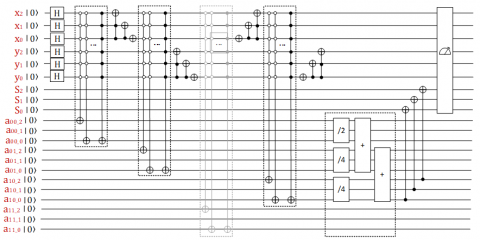Figure 22. Application of the convolution process for filter given in Figure 12.e. The gray dotted rectangle is not necessary for this filer. Total 21 qubits are required for 8x8 image and 2x2 filters

## 23.pngFigure 23. Convolution outputs for the quantum circuit given in Figure 22. The circuit runs for 512 shots

## 24.png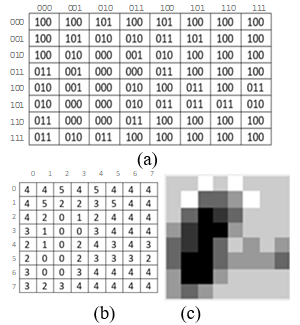Figure 24. Reconstruction of the convoluted image. a) Binary matrix form of results, b) decimal matrix, c) output image

Table 4. Simulation results for different input images

Original image

8x8, 3-qubit resolution

## 111.png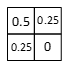## 222.png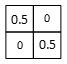## 333.png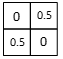## 444.png5. Conclusion

QC is exciting for many research areas with its computing power potential. On the other hand, window-based image applications such as DL need high computational power. But it is difficult to use QC in DL because the fundamentals of QC are different from traditional computing. Some variational circuits are proposed to apply quantum DL in the literature. However, the precision of these methods has not been validated in large input sets. There are also value-encoded methods for realizing window-based image processing applications. But they do not utilize the parallel processing capability of QC. In this study, a framework is proposed for the parallel processing of window-based quantum methods. In addition, convolution and pooling quantum circuits, which are the processes that require high computational power in DL, are implemented. To build these circuits, basic modules such as addition, multiplication, division, and comparison have been created. In this context, some modules such as x2, x4, /2, and /4 are implemented. Furthermore, a new approach for implementing the quantum comparison circuit is proposed. The created modules are run for all possible input pairs, and the results are validated. Because of the limitations of quantum simulators, and quantum computers in the NISQ era, the input images are reduced to 8x8 size and 3 qubit resolution. So, only 21 qubits are used, and the circuit length is kept at the appropriate depth for the efficient operation of quantum simulators. The generated circuits by the proposed methods are run on Qisqit aer_simulator. It is verified that the proposed methods successfully produce the expected results. Since the circuits are run in a simulation environment, satisfactory results could not be obtained in terms of running times. For the convolutional circuits, 512 shots were completed in an average of 8-10 minutes. However, it is thought that the real speed advantage of the methods will be observed with the emergence of gate-based quantum computers operating with low error rates in high volume, and with a sufficient number of qubits .

This study focused on the parallel application of the value-encoded convolution process in QC. Due to the limitations of quantum simulators, the sample input images are kept small. This study demonstrates how the convolution and pooling operations can be applied to QC with the traditional approach. With the proposed method, circuits that produce exactly the same result as the classical convolution process in the quantum computing environment are produced. With the use of the generated circuits, proven original deep learning steps are performed with high accuracy. However, many gates are required for encoding data and generating modules. The need for the use of large amounts of gates makes it difficult to implement on quantum computers in the NISQ era. On the other hand, it is possible to implement window-based image processing applications in parallel thanks to the proposed framework. The proposed framework is suitable for both value-based and variational circuits. In future studies, studies will be carried out to develop reversible circuit optimization methods so that the produced circuits can be created with fewer gates. In this way, the method will be carried out with fewer quantum gates and its applicability will be increased.

The highlights of the study can be summarized as below:

• Value-based convolution operations are simulated in parallel across an entire image with the help of the proposed framework.

• In contrast to variational quantum circuits, the classical data is encoded into quantum data with their exact values. So, the study guarantees that the results will be the same as the results obtained with the proven DL networks.

• A new approach, which uses fewer qubits and gates, is proposed to compare two binary numbers.

• Circuits for pooling are proposed with the help of modules that perform the ordering of two numbers. The circuits contain controlled swap and comparison modules.

• By repeating the patterns in module circuits, the basic modules with different qubit numbers can be obtained. And other convolutional and pooling circuits as needed can be easily implemented.

Acknowledgment

This study was supported by the TUBITAK (The Scientific and Technological Research Council of Turkey) under Grant No: 121E439.

This article work was carried out within the scope of Hasan Yetiş’s doctoral dissertation named "Development of Artificial Intelligence Algorithms Based on Quantum Computing". The supervisor of the thesis, Mehmet Karaköse.

References

 Kuutti, S., Bowden, R., Jin, Y., Barber, P., Fallah, S. (2021). A survey of deep learning applications to autonomous vehicle control. IEEE Transactions on Intelligent Transportation Systems, 22(2): 712-733. https://doi.org/10.1109/TITS.2019.2962338

 Floridi, L., Chiriatti, M. (2020). GPT-3: Its nature, scope, limits, consequences. Minds & Machines, 30(4): 681-694. https://doi.org/10.1007/s11023-020-09548-1

 Dale, R. (2021). GPT-3: What’s it good for? Natural Language Engineering., 27(1): 113-118. https://doi.org/10.1017/S1351324920000601

 Shahinfar, S., Meek, P., Falzon, G. (2020). ‘How many images do I need?’ Understanding how sample size per class affects deep learning model performance metrics for balanced designs in autonomous wildlife monitoring. Ecological Informatics, 57. https://doi.org/10.1016/j.ecoinf.2020.101085

 Maheshwaram, S. (2021). Future directions and challenges of deep learning. International Journal of Multi Disciplinary Research in Science, Engineering and Technology, 4(1). https://doi.org/10.15680/IJMRSET.2021.0401003

 Peemen, M., Mesman, B., Corporaal, H. (2011). Efficiency optimization of trainable feature extractors for a consumer platform. Advanced Concepts for Intelligent Vision Systems, 6915(1): 293-304. https://doi.org/10.1007/978-3-642-23687-7_27

 Tan, Z., Karakose, M. (2020). Comparative study for deep reinforcement learning with CNN, RNN, and LSTM in autonomous navigation. International Conference on Data Analytics for Business and Industry (ICDABI), Bahrain. https://doi.org/10.1109/ICDABI51230.2020.9325622

 Cui, Z., Ke, R., Pu, Z., Wang, Y. (2018). Stacked bidirectional and unidirectional LSTM recurrent neural network for network-wide traffic speed prediction. arXiv:1801.02143.

 Shimizu, T. (2020). Supercomputer Fugaku: Co-designed with application developers/researchers. IEEE Asian Solid-State Circuits Conference (A-SSCC), Hiroshima, Japan.

 Kodama, Y., Odajima, T., Arima, E., Sato, M. (2020). Evaluation of power management control on the supercomputer Fugaku. IEEE International Conference on Cluster Computing, pp. 484-493. https://doi.org/10.1109/CLUSTER49012.2020.00069

 Elsayed, N., Maida, S.A., Bayoumi, M. (2019). A review of quantum computer energy efficiency. IEEE Green Technologies Conference, Lafayette, LA, USA.

 Arute, F., Arya, K., Babbush, R. (2019). Quantum supremacy using a programmable superconducting processor. Nature, 574(7779): 505-510. https://doi.org/10.1038/s41586-019-1666-5

 Garg, S., Ramakrishnan, G. (2020). Advances in quantum deep learning: An overview. arXiv:2005.04316 [quant-ph].

 Benedetti, M., Lloyd, E., Sack, S., Fiorentini, M. (2019). Parameterized quantum circuits as machine learning models. Quantum Science and Technology, 4(4): 043001. https://doi.org/10.1088/2058-9565/ab4eb5

 Biamonte, J., Wittek, P., Pancotti, N., Rebentrost, P., Wiebe, N., Lloyd, S. (2017). Quantum machine learning. Nature, 549(7671): 195-202. https:/doi.org/10.1038/nature23474

 Adcock, J., Allen, E., Day, M., Frick, S. (2015). Advances in quantum machine learning. arXiv:1512.02900 [quant-ph].

 Yetiş, H., Karaköse, M. (2021). Obtaining Quantum Gate Models from Known Input and Output Values. Interdisciplinary Research in Technology and Management, CRC Press, 393-398.

 Matteo, O.D., Mosca, M. (2016). Parallelizing quantum circuit synthesis. Quantum Science and Technology, 1(1): 015003. https://doi.org/10.1088/2058-9565/1/1/015003

 Adedoyin, A., Ambrosiano, J., Anisimov, P. (2018). Quantum algorithm implementations for beginners. arXiv:1804.03719.

 Cross, A.W., Bishop, L.S., Smolin, J.A., Gambetta, J.M. (2017). Open Quantum Assembly Language. arXiv:1707.03429.

 Deutsch, D., Jozsa, R. (1992). Rapid solution of problems by quantum computation. Proceedings of the Royal Society of London. Series A: Mathematical and Physical Sciences, 439(1): 553-558. https://doi.org/10.1098/rspa.1992.0167

 Shor, P.W. (1994). Algorithms for quantum computation: Discrete logarithms and factoring. IEEE Proceedings 35th Annual Symposium on Foundations of Computer Science, 124-134.

 Grover, L.K. (1996). A fast quantum mechanical algorithm for database search. Proceedings of the Twenty-Eighth Annual ACM Symposium on Theory of Computing, pp. 212-219. https://doi.org/10.1145/237814.237866

 National Academies of Sciences Engineering and Medicine. (2019). Domestic Manufacturing Capabilities for Critical DoD Applications: Emerging Needs in Quantum-Enabled Systems: Proceedings of a Workshop. Washington, DC: The National Academies Press.

 Zhang, Y., Ni, Q. (2020). Recent advances in quantum machine learning. Quantum Engineering, 2(1): e34. https://doi.org/10.1002/que2.34

 Dunjko, V., Taylor, J.M., Briegel, H.J. (2016). Quantum-enhanced machine learning. Physical Review Letters, 117(13): 130501. https://doi.org/10.1103/PhysRevLett.117.130501

 Ciliberto, V., Herbster, M., Davide, A., Pontil, M., Severini, S. (2018). Quantum machine learning: A classical perspective. Proceedings of the Royal Society A: Mathematical, Physical and Engineering Sciences, 474(2209): 20170551. https://doi.org/10.1098/rspa.2017.0551

 Saini, S., Khosla, P., Kaur, M., Singh, G. (2020). Quantum driven machine learning. International Journal of Theoretical Physics, 59(12): 4013-4024. https://doi.org/10.1007/s10773-020-04656-1

 Tacchino, F., Macchiavello, C., Gerace, D., Bajoni, D. (2019). An artificial neuron implemented on an actual quantum processor. npj Quantum Information, 5(1): 26. https://doi.org/10.1038/s41534-019-0140-4

 Schuld, M., Sinayskiy, I., Petruccione, F. (2015). Simulating a perceptron on a quantum computer. Physics Letters A, 379(7): 660-663. https:/doi.org/10.1016/j.physleta.2014.11.061

 de Paula Neto, F.M., Filho, G.I.S., Monteiro, C.A. (2020). Approaches to avoid overfitting in a quantum perceptron. International Joint Conference on Neural Networks, UK.

 Tacchino, F., Barkoutsos, P., Macchiavello, C., Tavernelli, I., Gerace, D., Bajoni, D. (2020). Quantum implementation of an artificial feed-forward neural network. Quantum Science and Technology, 5(4). https:/doi.org/10.1088/2058-9565/abb8e4

 Parthasarathy, R., Bhowmik, R.T. (2021). Quantum optical convolutional neural network: A novel image recognition framework for quantum computing. IEEE Access, 9. https:/doi.org/10.1109/ACCESS.2021.3098775

 Oh, S., Choi, J., Kim, J.K., Kim, J. (2021). Quantum convolutional neural network for resource-efficient image classification: A Quantum Random Access Memory (QRAM) approach. International Conference on Information Networking (ICOIN), 50-52, Jeju Island, Korea (South). https:/doi.org/10.1109/ICOIN50884.2021.9333906

 Broughton, M. (2020). TensorFlow quantum: A software framework for quantum machine learning. arXiv:2003.02989 [cond-mat, physics:quant-ph].

 Chen, Y.C.S., Yang, H.C.H., Qi, J., Chen, P.Y., Ma, X., Goan, H.S. (2020). Variational quantum circuits for deep reinforcement learning. IEEE Access, 8(1): 141007-141024. https:/doi.org/10.1109/ACCESS.2020.3010470

 Kerenidis, I., Landman, J., Prakash, A. (2019). Quantum algorithms for deep convolutional neural network. arXiv:1911.01117.

 Bisarya, A., El Maouaki, W., Mukhopadhyay, S., Mishra, N. (2020). Breast cancer detection using quantum convolutional neural networks: A demonstration on a quantum computer. Oncology. https://doi.org/10.1101/2020.06.21.20136655

 Oh, S., Choi, J., Kim, J. (2020). A tutorial on quantum convolutional neural networks. arXiv:2009.09423.

 Yetiş, H., Karaköse, M. (2020). Quantum circuits for binary convolution. IEEE International Conference on Data Analytics for Business and Industry. https:/doi.org/10.1109/ICDABI51230.2020.9325659

 Cong, I., Choi, S., Lukin, D.M. (2019). Quantum convolutional neural networks. Nat. Phys., 15(12): 1273-1278. https://doi.org/10.1038/s41567-019-0648-8

 Su, J., Guo, X., Liu, C., Li, L. (2020). A new trend of quantum image representations. IEEE Access, 8(1): 214520-214537. https:/doi.org/10.1109/ACCESS.2020.3039996

 Zhang, Y., Lu, K., Gao, Y., Wang, M. (2013). NEQR: A novel enhanced quantum representation of digital images. Quantum Inf Process, 12(8): 2833-2860. https://doi.org/10.1007/s11128-013-0567-z

 Ma, Y., Ma, H., Chu, P. (2020). Demonstration of quantum image edge extraction enhancement through improved Sobel operator. IEEE Access, 8(1): 210277-210285. https:/doi.org/10.1109/ACCESS.2020.3038891

 Thapliyal, H., Ranganathan, N. (2013). Design of efficient reversible logic-based binary and BCD adder circuits. ACM Journal on Emerging Technologies in Computing Systems, 9(3): 1-31. https://doi.org/10.1145/2491682

 Li, P., Shi, T., Lu, A., Wang, B. (2019). Quantum circuit design for several morphological image processing methods. Quantum Information Processing, 18(12): 364. https://doi.org/10.1007/s11128-019-2479-z

 Wang, D. (2012). Design of quantum comparator based on extended general Toffoli gates with multiple targets. Computer Science, 39(9): 302-306.

 Foulds, S., Kendon, V., Spiller, T. (2021). The controlled SWAP test for determining quantum entanglement. Quantum Science and Technology, 6(3): 035002. https://doi.org/10.1088/2058-9565/abe458

 Jiang, S., Zhou, R.G., Hu, W., Li, Y. (2019). Improved quantum image median filtering in the spatial domain. International Journal of Theoretical Physics, 58(7): 2115-2133. https://doi.org/10.1007/s10773-019-04103-w

 Leymann, F., Barzen, J. (2020). The bitter truth about gate-based quantum algorithms in the NISQ era. Quantum Science and Technology, 5(4): 044007. https://doi.org/10.1088/2058-9565/abae7d

 Yetis, H., Karakose, M. (2021). Investigation of noise effects for different quantum computing architectures in IBM-Q at NISQ level. 25th International Conference on Information Technology (IT), Zabljak, Montenegro.